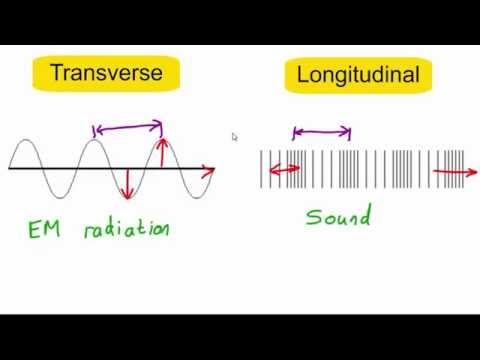### Main Difference

In the field of physics, we define a wave as an oscillation that is accompanied by a transfer of energy that travels through a medium (mass or space) with the frequency. Waves are classified into two major categories, longitudinal wave, and transverse wave. A longitudinal wave is that type of wave that moves in the direction of its propagation; its movement is often like vibrations. In this type of wave, the motion of the medium is from left to right or right to left on a common line, and the motion of the wave is to left or right. The best example of a longitudinal wave is sound waves. A transverse wave is that type of wave that moves at right angles to the direction of its propagation; its movement is like vibrations or flow. In this type of wave, the motion of the medium is up and down or down and up in a common line, and the motion of the wave is to the left or right. A common example of a transverse wave is a wave in water.

### Comparison Chart

 Longitudinal Wave Transverse Wave Longitudinal means running in the lengthwise. A longitudinal wave is a type of wave that moves in the direction of its propagation. Transverse means crosswise or extending across something. A transverse wave is that type of wave that moves at right angles to the direction of its propagation. Movement In a longitudinal wave, the wave moves to left or to the right, and the medium moves left and right. In a transverse wave, the wave moves to left or to the right and the medium moves up and down. Production Longitudinal waves are often produced due to vibrations (as in sound). Transverse has different phenomena. Example An example of a longitudinal wave is a sound wave. An example of a transverse wave is water waves.

### What is a Longitudinal Wave?

Longitudinal means running in the lengthwise. A longitudinal wave is that type of wave that moves in the direction of its propagation; its movement is often like vibrations. In this type of wave, the motion of the medium is from left to right or right to left on a common line, and the motion of the wave is to left or right.

An example of longitudinal waves is sound waves etc. A longitudinal wave is classified further into two as, longitudinal wave 1 and longitudinal wave 2. Longitudinal wave 1 is the wave in which a particle or object moves in a horizontal direction and shows a simple harmonic motion to the left or to the right.

An example is taken as when spring is stretched, its particle moves in the direction of stretch, the spring as a whole are not moving, it will vibrate as the wave pulse pass through it. In longitudinal wave 2, the motion is said as a disturbance moving parallel to the point of its propagation. In this many small particles move like simple harmonic motion to left or to the right. If spring is taken as an example, longitudinal wave 2 produces disturbance overall in the spring.

### What is the Transverse Wave?

Transverse means crosswise or extending across something. A transverse wave is that type of wave that moves at right angles to the direction of its propagation; its movement is like vibrations or flow. In this type of wave, the motion of the medium is up and down or down and up in a common line, and the motion of the wave is to the left or right. A common example of a transverse wave is a wave in water etc.

A transverse wave is also classified into two as, transverse wave 1 and transverse wave 2. Transverse wave 1 is the wave in which a particle or object moves in a vertical direction and show a simple harmonic motion to up or down. For example, when you are shaking a rope, imagine one of its particles moving in the waveform. Transverse wave 2, in this all the particles of the objects move in the medium and produce a wave. The direction of the wave is to left or to the right from the point of propagation, and the movement of the medium is up and down.

### Key Differences

1. Longitudinal means running in the lengthwise. A longitudinal wave is that type of wave that moves in the direction of its propagation, whereas transverse means crosswise or extending across something. A transverse wave is that type of wave that moves at right angles to the direction of its propagation.
2. In a longitudinal wave, the wave moves to left or to the right, and the medium moves left and right whereas, in a transverse wave, the wave moves to left or to the right and the medium moves up and down.
3. Longitudinal waves are often produced due to vibrations (as in sound) whereas, transverse has different phenomena.
4. An example of a longitudinal wave is a sound wave, while an example of a transverse wave is water waves.